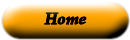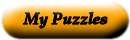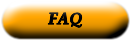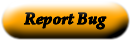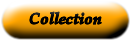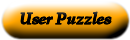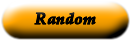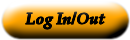### MATH VOCAB (GREEN)

RENEE EARL

 1 decimal point _____ Two lines that cross over each other 2 difference _____ Numbers multiplied together 3 place value _____ The value of a digit based upon the place of the digit 4 product _____ A number that uses place value and a decimal point to show 5 complimentary angle _____ Answer to an addition problem 6 intersecting lines _____ Two angles that have a sum that equals 90 degrees 7 perpendicular _____ To rewrite a whole number to the nearest ten, hundred, 8 protractor _____ Answer to a subtraction problem 9 rounding _____ A number that has only two factors 10 decimal _____ The third place value to the right of the decimal point 11 sum _____ An instrument that measures angles. 12 thousandths _____ A dot used to separate the ones and tenths place value 13 composite number _____ A number with three or more factors 14 factors _____ Answer to a multiplication problem 15 prime number _____ Two lines intersecting and making right angles.

Use the "Printable HTML" button to get a clean page, in either HTML or PDF, that you can use your browser's print button to print. This page won't have buttons or ads, just your puzzle. The PDF format allows the web site to know how large a printer page is, and the fonts are scaled to fill the page. The PDF takes awhile to generate. Don't panic!Web armoredpenguin.com